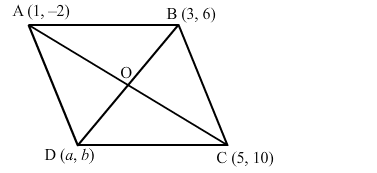# If three consecutive vertices of a parallelogram ABCD are A(1, −2), B(3, 6) and C(5, 10), find its fourth vertex D.

Question:

If three consecutive vertices of a parallelogram ABCD are A(1, −2), B(3, 6) and C(5, 10), find its fourth vertex D.

Solution:

Let A(1, −2), B(3, 6) and C(5, 10) be the three vertices of a parallelogram ABCD and the fourth vertex be D(a, b).
Join AC and BD intersecting at O.We know that the diagonals of a parallelogram bisect each other.
Therefore, O is the midpoint of AC as well as BD.

Midpoint of $A C=\left(\frac{1+5}{2}, \frac{-2+10}{2}\right)=\left(\frac{6}{2}, \frac{8}{2}\right)=(3,4)$

Midpoint of $B D=\left(\frac{3+a}{2}, \frac{6+b}{2}\right)$

Therefore, $\frac{3+a}{2}=3$ and $\frac{6+b}{2}=4$

$\Rightarrow 3+a=6$ and $6+b=8$

$\Rightarrow a=6-3$ and $b=8-6$

$\Rightarrow a=3$ and $b=2$

Therefore, the fourth vertex is D(3, 2).